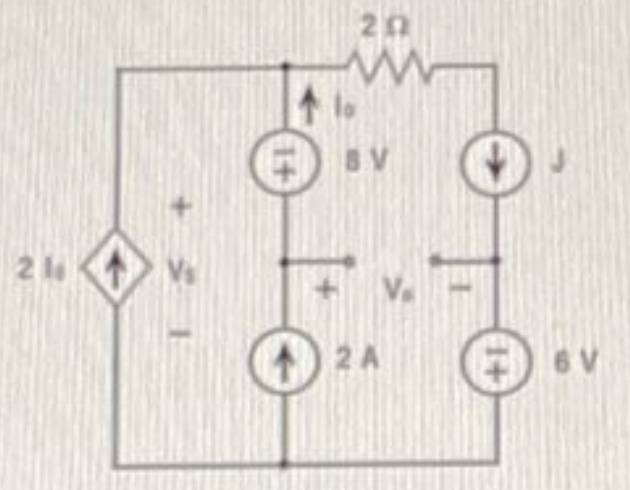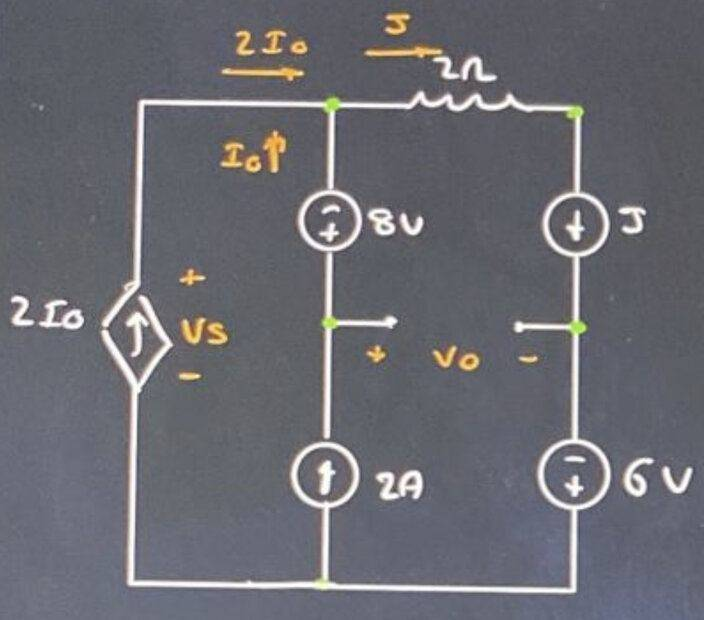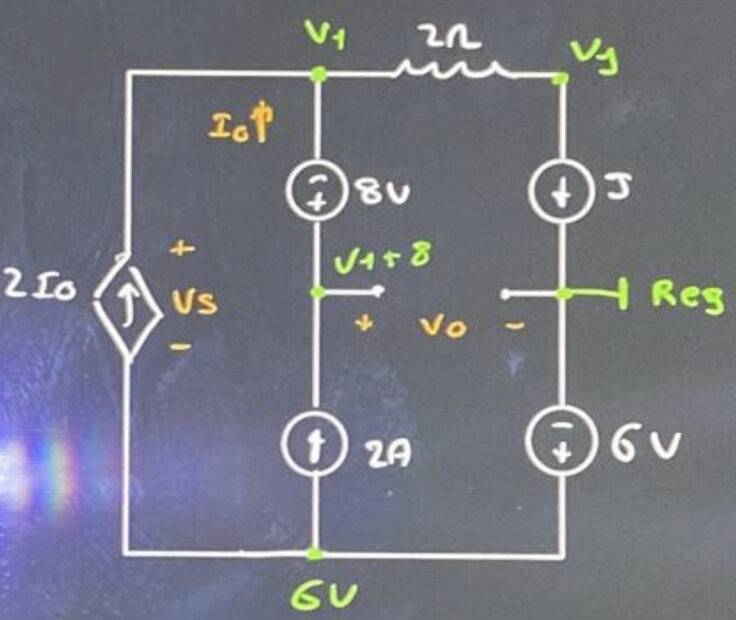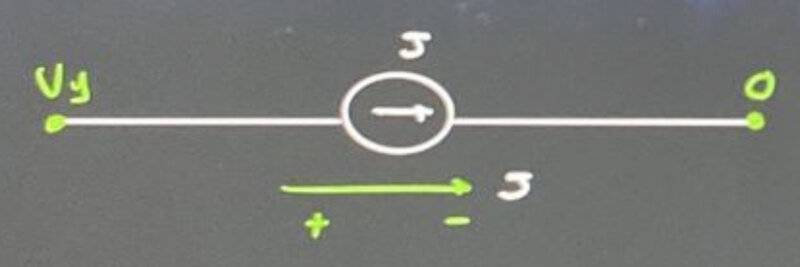# Power generated by this current source

• Guillem_dlc

#### Guillem_dlc

Homework Statement
In the circuit of the figure knowing that ##V_0=10\, \textrm{V}## determine ##V_S## and the power generated by the source ##J##.

Sol: ##V_S=-4\, \textrm{V}##, ##P_J=60\, \textrm{W}##
Relevant Equations
Kirchoff law
Figure:My attempt at a solution:1st kirchoff law:
$$J=2I_0+I_0=6\, \textrm{A}$$$$V_1+8=10\rightarrow \boxed{V_1=2}$$
$$V_1=6-V_S\rightarrow \boxed{V_S=-4\, \textrm{V}}$$
We are looking for ##P## generated in ##J##
$$V_J=V_1-2\cdot J=2-2\cdot 6=-10\, \textrm{V}$$$$\boxed{P_{\textrm{gen}}=(V_J-0)J=-10\cdot 6=-60\, \textrm{W}}$$
Wouldn't this exercise do like this? I have tried it but the power is negative.

#### Attachments

Your ##V_1=6-V_S## should be ##V_1=6+V_S##, but it looks like that was just a typo.
The rest looks right. I would not rule out the possibility that the answer is negative.

haruspex said:
Your ##V_1=6-V_S## should be ##V_1=6+V_S##, but it looks like that was just a typo.
The rest looks right. I would not rule out the possibility that the answer is negative.
Is it negative?

Guillem_dlc said:
Is it negative?
As I wrote, your working looks fine, apart from that typo. So unless I am missing something the answer is negative.

•Guillem_dlc